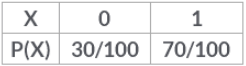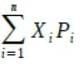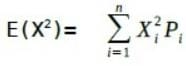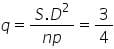JEE  >  Test: Binomial Distribution

# Test: Binomial Distribution

Test Description

## 10 Questions MCQ Test Mathematics (Maths) Class 12 | Test: Binomial Distribution

Test: Binomial Distribution for JEE 2023 is part of Mathematics (Maths) Class 12 preparation. The Test: Binomial Distribution questions and answers have been prepared according to the JEE exam syllabus.The Test: Binomial Distribution MCQs are made for JEE 2023 Exam. Find important definitions, questions, notes, meanings, examples, exercises, MCQs and online tests for Test: Binomial Distribution below.
Solutions of Test: Binomial Distribution questions in English are available as part of our Mathematics (Maths) Class 12 for JEE & Test: Binomial Distribution solutions in Hindi for Mathematics (Maths) Class 12 course. Download more important topics, notes, lectures and mock test series for JEE Exam by signing up for free. Attempt Test: Binomial Distribution | 10 questions in 10 minutes | Mock test for JEE preparation | Free important questions MCQ to study Mathematics (Maths) Class 12 for JEE Exam | Download free PDF with solutions
 1 Crore+ students have signed up on EduRev. Have you?
Test: Binomial Distribution - Question 1

### The variance of the distribution is​

Detailed Solution for Test: Binomial Distribution - Question 1

Mean= E(X) == 0×0.3+1×0.7
=0.7= 02×0.3+12×0.7 =0.7
Now, ∴ Var(X)= E(X2)-(E(X))2
= 0.7−(0.7)2
= 0.7 − 0.49
= 0.21

Test: Binomial Distribution - Question 2

### A die is tossed twice. Getting a number greater than 4 is considered a success. Then the variance of the probability distribution of the number of successes is:​

Detailed Solution for Test: Binomial Distribution - Question 2

In a single toss, P(success) = 2/6 = 1/3 and P(non-success) = (1 − 1/3) = 2/3.
P(X=0) = P(non-success in the 1st draw and non-success in the second)
(2/3 × 2/3) = 4/9.
P(X=1) = P(success in the 1st toss and non-success in the 2nd) or (non-success in the 1st toss and success in the 2nd)]
(1/3 x 2/3) + (2/3 × 1/3) = 4/9.

Test: Binomial Distribution - Question 3

### A man make attempts to hit the target. The probability of hitting the target is 3/5 . Then the probability that A hit the target exactly 2 times in 5 attempts is:​

Detailed Solution for Test: Binomial Distribution - Question 3

Probability of success(p) = ⅗
q = 1 - p
= 1 - (⅗)  ⇒ ⅖
Probability of 2 hit in 5 attempts = 5C2 (⅗)2 (⅖)3
= 144/625

Test: Binomial Distribution - Question 4

Trials of a random experiment are called Bernoulli trials, if they satisfy the condition/s:​

Test: Binomial Distribution - Question 5

In binomial probability distribution, mean is 3 and standard deviation is 3/2 . Then the probability distribution is:

Detailed Solution for Test: Binomial Distribution - Question 5

Mean = np = 3, S.D. (npq)½ = 3/2p = ¼            n = 12
Hence, binomial distribution is (p+q)n = (¾ + ¼)12

Test: Binomial Distribution - Question 6

A random variable is a real valued function whose domain is the.

Test: Binomial Distribution - Question 7

In a box containing 100 bulbs, 10 are defective. The probability that out of a sample of 5 bulbs, none is defective is​

Detailed Solution for Test: Binomial Distribution - Question 7

Probability of getting first non-defective bulb =90/100
Probablity of getting second non-defective bulb =89/99
Probablity of getting third non-defective bulb =88/98
Probablity of getting fourth non-defective bulb =87/97
Probablity of getting fifth non-defective bulb =86/96
So, probablity of getting all non-defective bulbs in a sample of 5 bulbs =
(90/100)∗(89/99)∗(88/98)∗(87/97)∗(86/96)
= closest option is b

Test: Binomial Distribution - Question 8

The mean and variance of a binomial distribution are 4 and 3 respectively, then the probability of getting exactly six successes in this distribution is:

Detailed Solution for Test: Binomial Distribution - Question 8

Let X˜B(n,p) be a binomial variate with mean 4 and variance 3. Then,
np=4,and npq=3
⇒q=3/4,p=1/4 and n=16
∴P(X=r)=.16Cr(1/4)r (3/4)(16−r) ,r=0,1,2,....,16
⇒P(X=6)= 16C6(1/4)6 ((3)/(4))10

Test: Binomial Distribution - Question 9

In a box of 10 electric bulbs, two are defective. Two bulbs are selected at random one after the other from the box. The first bulb is put back in the box before making the second selection. The probability that both the bulbs are not defective is:​

Test: Binomial Distribution - Question 10

Ten eggs are drawn successively with replacement from a lot containing 10% defective eggs. The probability that there is at least one defective egg is:

Detailed Solution for Test: Binomial Distribution - Question 10

Probability of an egg being defective =10/100=110
So, probability of an egg being non-defective=1−0.1=0.9
10 eggs are drawn successively with replacement.
So, the probability of getting no defective egg =(0.9)10
Hence, the probability that there is at least one defective egg = 1−(0.9)10

## Mathematics (Maths) Class 12

209 videos|218 docs|139 tests
 Use Code STAYHOME200 and get INR 200 additional OFF Use Coupon Code
Information about Test: Binomial Distribution Page
In this test you can find the Exam questions for Test: Binomial Distribution solved & explained in the simplest way possible. Besides giving Questions and answers for Test: Binomial Distribution , EduRev gives you an ample number of Online tests for practice

## Mathematics (Maths) Class 12

209 videos|218 docs|139 tests